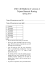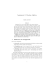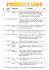# Beyond classical chip design Exercise III

## Transcription

Beyond classical chip design Exercise III
```Beyond classical chip design
Exercise III
summer term 2015
Matthias F¨
ugger
Ex 11.
Prove (A simulates B) → (B implements A).
Ex 12 & 13. We have shown that two joined wires are not a wire. In the slides
there were a circuit A with a fed-back OR gate with one wire in its feedback
and a circuit B that had two wires in its feedback. Assume that initially all
ports have value 0. (i) Does A implement B or vice versa? (ii) Does A simulate
B or vice versa?
Ex 14 & 15. Assume your combinational logic delay d (in ns) is distributed
according to Norm(1, σ) with 3σ = 0.2. What is the tradeoff between speed
versus reliability with a clockless and with a clocked approach? Let t(k), where
k ≥ 1, be the time the circuit produces its k th data item. For the synchronous
circuit t(k) = kT , where T is its clock period. For the clockless circuit t(k) =
t(k − 1) + dreq (k) + dack (k) where t(0) = 0 and dreq (k) and dack (k) for all k ≥ 1
are independently Norm(1, σ) distributed. Let P (fail ≤ k) be the probability
that a timing violation occurs by the k th data item in the synchronous circuit,
i.e., ddat (k 0 ) > T for some k 0 with 1 ≤ k 0 ≤ k.
(i) Compare the two design styles for several mission times (in terms of
data items). Assuming that the synchronous circuit is allowed to fail with
probability at most 10−9 during mission, for which mission times M would you
use which design style if you want high expected throughput (E(t(M )))? (ii)
What happens if you further decrease P (fail ≤ k) to e.g. 10−14 ?
```

### From the Desk of Dean G. De Vries Bock - ELCSA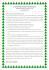### Vincent Camus### Why try Circuit?### Electricity Study Guide - Key What are series circuits?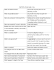### Assignment 1- Unit 35 Activity 1 P1 P2 P4 M1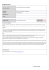### Meter Main with Load Center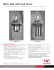### Electronics_exercises_files/extra 2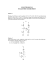### INF5481 RF Circuit, Theory and Design Assignment #3 Problem 1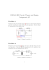### ac / dc electrical learning system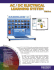### Exam#2 sol_A